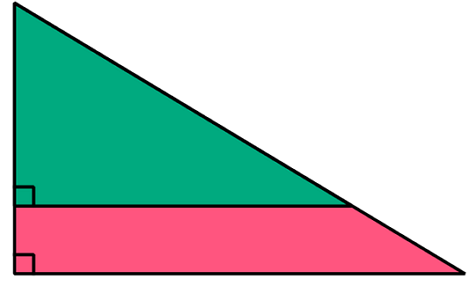# The Perimeter Is A Useful Information?

Geometry Level 3The above shows two similar right triangles. The side lengths of the green right triangles are 9, 12 and 15.

Given that both the perimeter of the green region and the pink region are equal, find the area of the entire figure (green and pink regions).

×